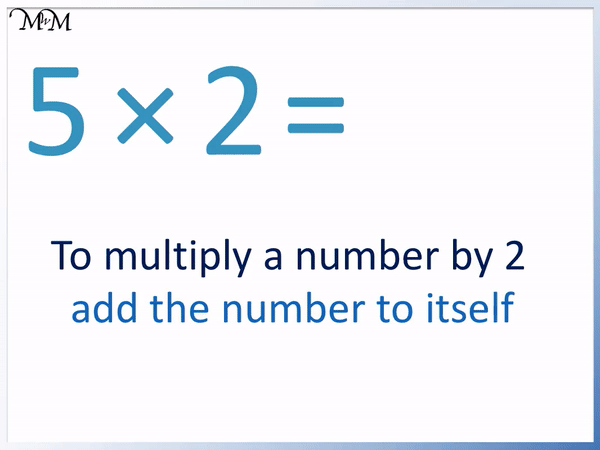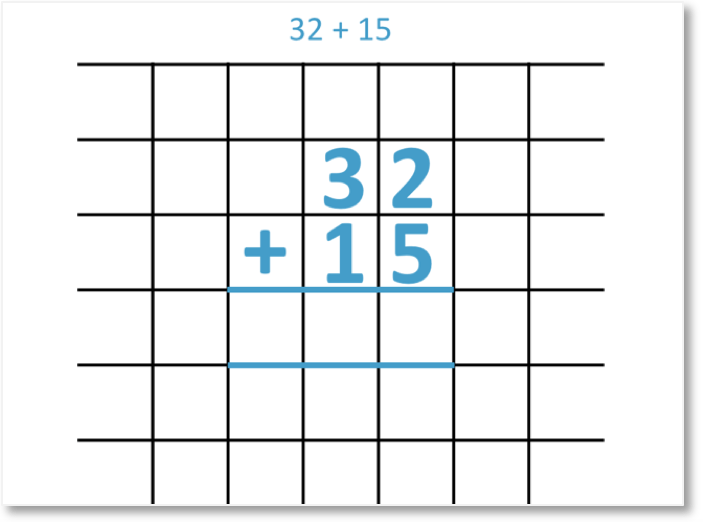# The 2 Times Table

The 2 Times TableThe 2 times table is:

• 1 × 2 = 2
• 2 × 2 = 4.
• 3 × 2 = 6
• 4 × 2 = 8
• 5 × 2 = 10
• 6 × 2 = 12
• 7 × 2 = 14
• 8 × 2 = 16
• 9 × 2 = 18
• 10 × 2 = 20
• 11 × 2 = 22
• 12 × 2 = 24• To multiply a number by two, simply double it.
• The easiest way to double a number is to add it on to itself.
• It helps to know addition before trying to learn the 2 times table.
• It is also useful to practise skip counting in twos before learning the 2 times table.
• We can see that numbers the 2 times table follow a pattern of ending in 2, 4, 6, 8 and 0 in that order.

To multiply a number by 2, add it to itself.

The 2 times table repeats the pattern of ending in 2, 4, 6, 8 and 0.• To multiply a number by 2, add the number to itself.
• To multiply 5 by 2, we add 5 to 5.
• 5 + 5 = 10 and so, 5 × 2 = 10.#### 2 Times Table Flashcards

Click on the 2 times table flashcards below to memorise the 2 times table.

×
×

=

# The 2 Times Table

## What is the 2 Times Table?

The 2 times table is:

• 1 × 2 = 2
• 2 × 2 = 4.
• 3 × 2 = 6
• 4 × 2 = 8
• 5 × 2 = 10
• 6 × 2 = 12
• 7 × 2 = 14
• 8 × 2 = 16
• 9 × 2 = 18
• 10 × 2 = 20
• 11 × 2 = 22
• 12 × 2 = 24

Here is the 2 times table shown in a poster:## How to Learn the 2 Times Table

To learn the 2 times table, double each number you are multiplying by 2. To double a number easily, simply add it on to itself. For example 3 × 2 can be found by working out 3 + 3, which is 6.

The 2 times table can be memorised since the numbers repeat the pattern of ending in 2, 4, 6, 8 and 0.Here is another example of multiplying by 2 by adding a number to itself.

Here we have 5 × 2.

We add 5 to itself. 5 + 5 = 10.

And therefore, 5 × 2 = 10.We can use the trick of adding a number to itself to work out all of the numbers in the two times table easily.We can see that the answers in the times table always end in 2, 4, 6, 8 and then 0.

This pattern repeats and can help us to work out the next number along if we know the previous times table.

For example if we are asked what 9 × 2 is, we might already remember that 8 × 2 = 16.

16 ends in 6 and we know that the pattern repeats 2, 4, 6, 8, 0. After 6 comes 8.

If 8 × 2 = 16, then the next times table along is 9 × 2 = 18. We just remember that 8 comes after 6 in our two times table pattern.Alternatively, we add 9 to itself. 9 + 9 = 18.

And therefore, 9 × 2 = 18.

## Teaching the 2 Times Table

When teaching the 2 times table, it is important to first be strong in addition. This is because to work out the two times table, we need to add the number on to itself.

It is also important to practise skip counting in twos. Knowing how to count on in twos helps us to go from one times table to the next by adding two to the answer.

It is also helpful to teach the 2 times table arranged in rows of 5 so that we can easily see the pattern of the numbers ending in 2, 4, 6, 8 and 0.

For example, below is the full 2 times table arranged in rows of 5.We can see that each column has numbers ending in 2, 4, 6, 8 and then 0.

After we have a number ending in zero, we simply move to the next column.

We can teach that every time we go from ending in 8 to ending in 0, we must increase the tens digit by 1.We add 2 to 4 to get 6.

We add 2 to 6 to get 8 and so on.

It is helpful for children to realise that they can just add 2 to the previous number to find out the next number in the 2 times table.

It is helpful to memorise the 2 times table by simply chanting through the answers by adding two each time.Now try our lesson on 2-Digit Column Addition where we learn how to use the column addition method for adding two numbers.